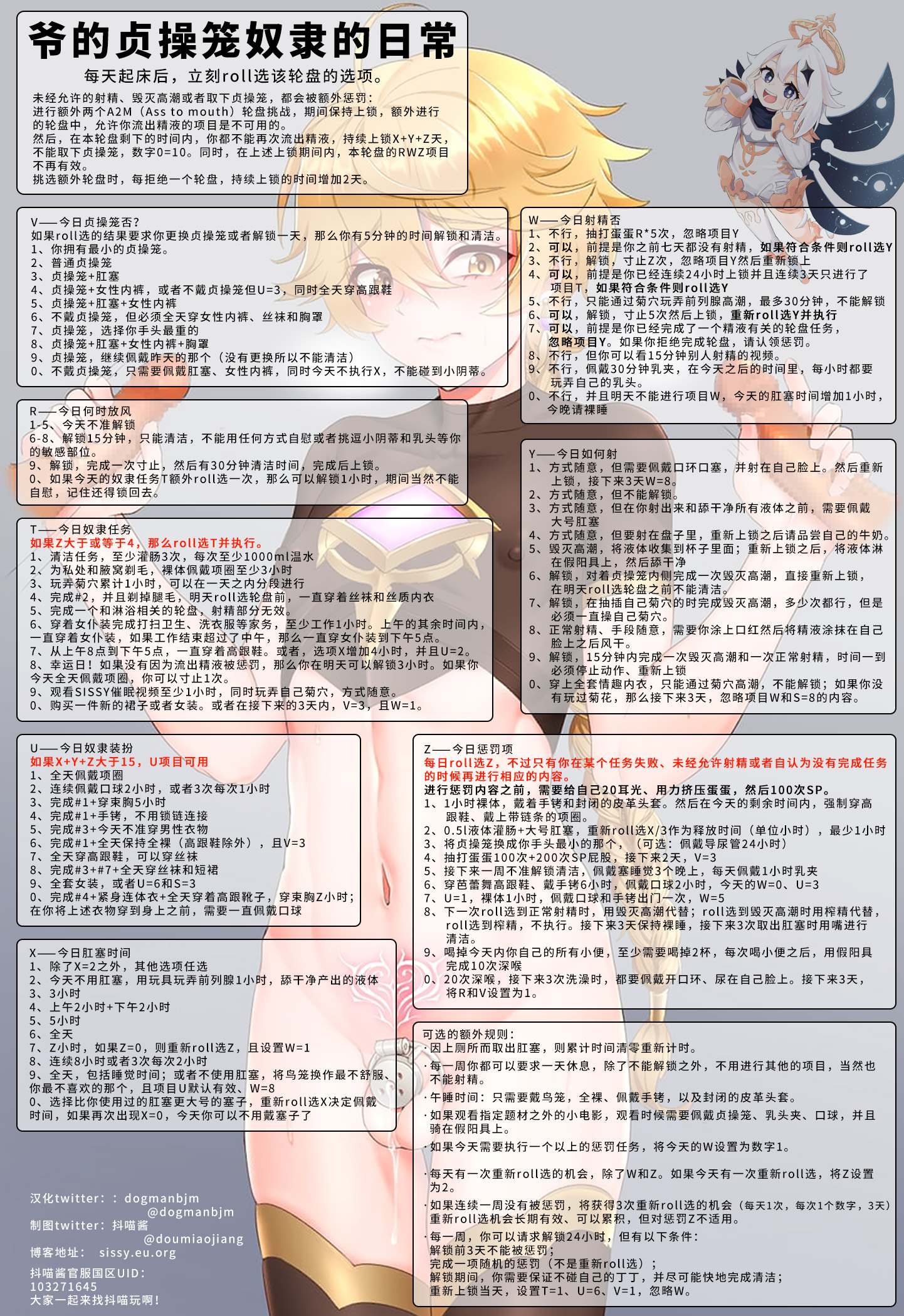R=1 S=0 T=0 U=6 V=0 W=0 X=1 Y=0 Z=5
**↑ ↑ ↑ ↑ ↑